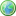Accessibility options:

# Simple linear equations resources

Show me all resources applicable to

### Video with captions which require edits (1)Simple Linear Equations
In this unit we give examples of simple linear equations and show you how these can be solved. In any equation there is an unknown quantity, x say, that we are trying to find. In a linear equation this unknown quantity will appear only as a multiple of x, and not as a function of x such as x2, x3, sin x and so on. Linear equations occur so frequently in the solution of other problems that a thorough understanding of them is essential. (Mathtutor Video Tutorial) The video is released under a Creative Commons license Attribution-Non-Commercial-No Derivative Works and the copyright is held by Skillbank Solutions Ltd.

Website design by Pink Mayhem, Leicester